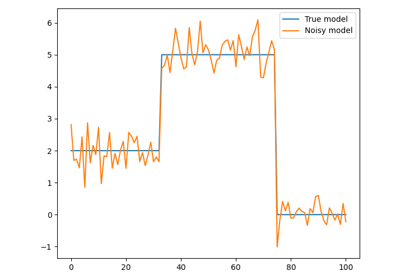# pyproximal.RelaxedMumfordShah#

class pyproximal.RelaxedMumfordShah(sigma=1.0, kappa=1.0)[source]#

Relaxed Mumford-Shah norm proximal operator.

Proximal operator of the relaxed Mumford-Shah norm: $$\text{rMS}(x) = \min (\alpha\Vert x\Vert_2^2, \kappa)$$.

Parameters
sigmafloat or list or np.ndarray or func, optional

Multiplicative coefficient of L2 norm that controls the smoothness of the solutuon. This can be a constant number, a list of values (for multidimensional inputs, acting on the second dimension) or a function that is called passing a counter which keeps track of how many times the prox method has been invoked before and returns a scalar (or a list of) sigma to be used.

kappafloat or list or np.ndarray or func, optional

Constant value in the rMS norm which essentially controls when the norm allows a jump. This can be a constant number, a list of values (for multidimensional inputs, acting on the second dimension) or a function that is called passing a counter which keeps track of how many times the prox method has been invoked before and returns a scalar (or a list of) kappa to be used.

Notes

The $$rMS$$ proximal operator is defined as :

$\begin{split}\text{prox}_{\tau \text{rMS}}(x) = \begin{cases} \frac{1}{1+2\tau\alpha}x & \text{ if } & \vert x\vert \leq \sqrt{\frac{\kappa}{\alpha}(1 + 2\tau\alpha)} \\ \kappa & \text{ else } \end{cases}.\end{split}$
1

Strekalovskiy, E., and D. Cremers, 2014, Real-time minimization of the piecewise smooth Mumford-Shah functional: European Conference on Computer Vision, 127–141.

Methods

 __init__([sigma, kappa]) affine_addition(v) Affine addition chain(g) Chain grad(x) Compute gradient postcomposition(sigma) Postcomposition precomposition(a, b) Precomposition prox(*args, **kwargs) proxdual(**kwargs)

## Examples using pyproximal.RelaxedMumfordShah#Relaxed Mumford-Shah regularization

Relaxed Mumford-Shah regularization